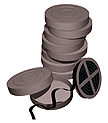# Stony Brook MathematicsVideo Archive### Courses

• MAP 103
Proficiency Algebra

• MAT 122
Overview of Calculus

• MAT 123
Introduction to Calculus

• MAT 125
Calculus A

• MAT 126
Calculus B

• MAT 127
Calculus C

• MAT 132
Calculus II

• MAT 307
Multivariable Calculus with Linear Algebra

• MAT 638